0
153

# Mental Ability Questions for SSC CGL PDF

Download SSC CGL Questions on Mental Ability  PDF based on previous papers very useful for SSC CGL exams. Mental Ability Questions for SSC exams.

Question 1: Select the option that is related to the third letter-cluster in the same way as the second letter-cluster is related to the first letter-cluster.
DFIM : WURN :: GENP : ?

a) SOLJ

b) TROK

c) TPNL

d) TPMK

Question 2: Select the number-pair in which the two numbers are related in the same way as are the two numbers of the following number-pair.
12 : 42

a) 15 : 52

b) 24 : 82

c) 8 : 28

d) 16 : 53

Question 3: Select the word-pair in which the two words are related in the same way as are the two words in the following word pair.
Caress : Affection

a) Crime : Confession

b) Interview : Selection

c) Anger : Emotion

d) Kick : Hostility

Instructions

In each of the following questions, find the odd number / letters /word from the given alternatives.

Question 4:

a) 162

b) 405

c) 567

d) 644

Question 5: Select the odd word/letters/number/number pair from the given alternatives.

a) 17

b) 23

c) 29

d) 33

Instructions

In the following two questions, which one set of letters when sequentially placed at the gaps in the given letter series shall complete it ?

Question 6: a_ _ dba _ _ bcad _ _ da _ _cd

a) aabbccdd

b) bccdbcab

c) abcddcba

d) cbcddcbb

Instructions

In each of the following questions, select the missing number from the given responses.

Question 7: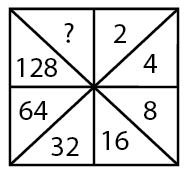a) 240

b) 246

c) 250

d) 256

Instructions

In each of the following questions, a series is given, with one term missing. Choose the correct alternative from the given ones that will complete the series.

Question 8: 1, 2, 2, 4, 3, 8, 7, 10, ?

a) 11

b) 13

c) 9

d) 8

Instructions

From the given answer figures, select the one in which the question figure is hidden/embedded.

Question 9: If a mirror is placed on the line MN, then which of the answer figures is the correct image of the given question figure? Question Figure :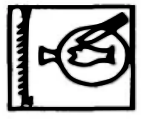a)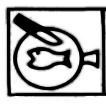b)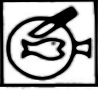c)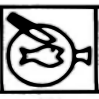d)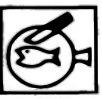Question 10: A piece of paper is folded and cut as shown below in the question figures. From the given answer figures, indicate how it will appear when opened. Questions Figure :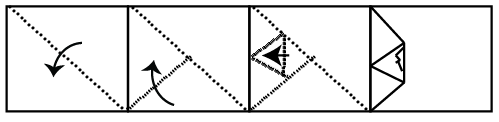a)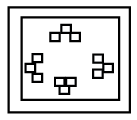b)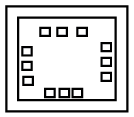c)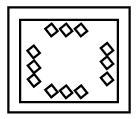d)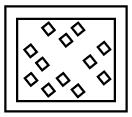Question 11: Arrange the given words in the sequence in which they occur in the dictionary.
i. Foresay
ii. Foreseize
iii. Foreseeable
iv. Foresail

a) i, iii, iv, ii

b) i, iii, ii, iv

c) iii, i, ii, iv

d) iv, i, iii, ii

Question 12: Arrange the given words in the sequence in which they occur in the dictionary.
ii.Treason
iii. Treacherous

a) ii, iii, iv, i

b) iii, iv, ii, i

c) iii, iv, i, ii

d) i, ii, iii, iv

Instructions

In the following questions, which one of the given responses would be a meaningful order of the following?

Question 13: 1. Frog
2. Eagle
3. Grasshopper
4. Snake
5. Grass

a) 5, 3, 4, 2, 1

b) 1, 3, 5, 2, 4

c) 5, 3, 1, 4, 2

d) 3, 4, 2, 5, 1

Question 14: Which one of following responses would be a meaningful order of the following ?
1. Chapter
2. Index
3. Bibliography
4. Introduction
5. Preface

a) 2, 4, 1, 3, 5

b) 4, 2, 5, 1, 3

c) 5, 2, 4, 1,3

d) 5, 1, 4, 3, 2

Question 15: Introducing a boy, a girl said, “He is the son of the sister of my mother’s brother”. How is that boy related to that girl?

a) Cousin

b) Uncle

c) Father-in-law

d) Niece

Apart from 644, all other given numbers are multiples of 9.

=> Ans – (D)

Apart from 33, all are prime numbers, hence 33 which is a composite number is the odd one out.

=> Ans – (D)

The pattern is that, in a group of 4 letters, the combination of first four alphabets is used.

=> abcd bacd bcad bcda abcd

=> Ans – (B)let the missing number be y

here we can see as we start moving in clock wise direction from “2” we will realize that every time the number is getting doubled and hence in the missing box we will fill double of 128 which is 256 and so , y = 256

It is a combination of 2 series, one at odd places and other at even places.

1) : 1,2,3,7, 11,12,13,17     (Difference is 1,1,4,1,1,4,…)

2) : 2,4,8,10, 14,16,20        (Difference is 2,4,2,4,2,….)

=> Ans – (A) : 11

In the above figure, fish is facing west, => in the mirror it will face east.

=> (A) & (D) are eliminated.

Also, knife is facing south-west, thus in the mirror it will face south-east

=> (B) is also eliminated.

Ans – (C)

As per the order of dictionary :

= Foresail -> Foresay -> Foreseeable -> Foreseize

$\equiv$ iv, i, iii, ii

=> Ans – (D)

As per the order of dictionary :

$\equiv$ iii, iv, i, ii

=> Ans – (C)

The correct order of a food chain is :

= Grass -> Grasshopper -> Frog -> Snake -> Eagle

$\equiv$ 5, 3, 1, 4, 2

=> Ans – (C)

The correct order in a book is :

= Preface -> Index -> Introduction -> Chapter -> Bibliography

$\equiv$ 5, 2, 4, 1,3

=> Ans – (C)